| by Arround The Web | No comments

## Python Count Characters in String

In Python, there can be situations where we need to count the characters in a string for data analysis, text processing, or other applications. This is a common approach that can be accomplished with several Python methods. These include the following:

## How to Count the Characters in a Python String?

To count the characters in a string in Python, the following approaches can be used:

## Method 1: Count the Characters in a String in Python Using the “len()” Function

The most straightforward way to count characters in a string is to use the built-in “len()” function. The “len()” function retrieves the character count of a particular string.

Syntax

len(object)

In the above syntax, “object” refers to the object of which we have to find the length, such as a string, list, tuple, etc.

Example

Here is an example code:

string_value = "Python Guide"
count = len(string_value)
print(count)

In the above code, the “len()” function takes the initialized string value as an argument and retrieves the total count of the characters in the string.

Output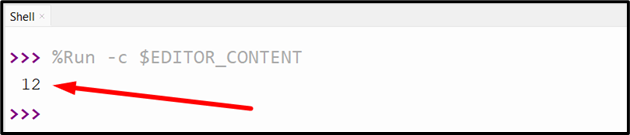The above outcomes show that the total count of the given string is “12”.

## Method 2: Count the Characters in a String in Python Using the “Counter” Class

The “Counter” class from the “collections” module is a powerful tool for counting elements in a list or a string.

Syntax

Counter(iterable_or_mapping)

In this syntax, “iterable_or_mapping” corresponds to an optional parameter that can be a sequence, a dictionary with keys and counts, or keyword arguments that map string names to counts.

Example

Overview of the following lines of code:

from collections import Counter
string_value = "Python Guide"
count = Counter(string_value)
print(sum(count.values()))

In the above code block:

• The “Counter” class is imported from the “collections” module and the string is initialized, respectively.
• In the next step, the “Counter()” function accepts the initialized string as an argument and returns a dictionary with the counts of each element in the iterable.
• Lastly, the combined “values()” and “sum()” functions fetch the total count of the characters in the given string.

OutputThe above output shows that the total count of the given string is “12”.

## Method 3: Count the Characters in a String in Python Using “Dictionary Comprehension”

Dictionary Comprehension” is a simple Python technique for creating dictionaries. This approach can also be utilized to count characters in a string.

Syntax

{key: value for key, value in iterable if condition}

Example

Below is an example code:

string_value = "Python, Java, C++ Guide"
count = {i: string_value.count(i) for i in set(string_value)}
print(sum(count.values()))

In the above code snippet:

• The “Dictionary Comprehension” is used along with the “for” loop and “set()” function to count each element in the iterable.
• After that, the “values()” and “sum()” functions are applied to get the total count of the characters in the given string from the dictionary.

Output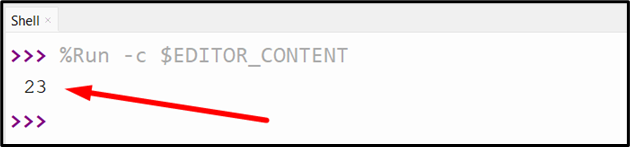Based on the above results, the total count of the string is “23“.

## Method 4: Count the Characters in a String in Python Using “for” Loop

The “for” loop is used to iterate through each string character and count the occurrences of each character.

Example

Let’s go through the following code:

string_value = "Welcome to Python Guide"
count = 0
for i in string_value:
count += 1
print(count)

In the above code:

• Firstly, the “string” is initialized, and the “count” is set to “0”.
• Now, apply the “for” loop to iterate over the string and count the total characters in the string via incrementing the count.

OutputAccording to the above results, the initialized string has a total count of “23“.

## Method 5: Count the Characters in a String in Python Using the “Lambda” Function

The “Lambda” functions are small and anonymous that can be used for simple tasks. Additionally, we can utilize a lambda function to count the string characters.

Syntax

lambda parameters: expression

Example

Let’s overview the following code:

string_value = "Welcome to Python Tutorial"
count = (lambda x: len(x))(string_value)
print(count)

In this code, the “lambda” function is used along with the “len()” function to get the total count of the characters in the given string.

Output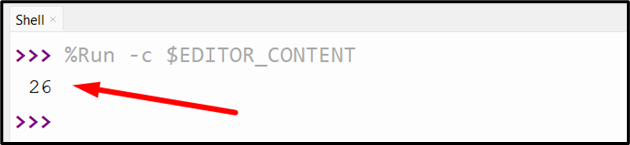According to the above results, the string has a total count of “26“.

## Method 6: Count the Characters in a String in Python Using “Regex”

Regular expressions” or “regex” is a powerful tool for pattern matching in Python. We can use the regex “re.findall()” function along with the “len()” function to count the occurrences of characters in a string.

Syntax

re.findall(pattern, string, flags=0)

In the above syntax:

• pattern” is a regular expression that defines the search criteria.
• string” is the text/word that needs to be searched.
• flags” are optional modifiers that affect how the search is performed.

Example

Here is an example code:

import re
string_value = "Python Tutorial"
count = len(re.findall(".", string_value))
print(count)

According to the above code:

• The “findall()” function of the “re” module is used to find all matches of the pattern in the string value and returns a list of matched characters.
• The pattern “.” is used in the “re.findall()” function to match any character except the new line.
• The “len()” function is used to get the total count of the characters in the string by accepting the value returned by the “re.findall()” function.

Output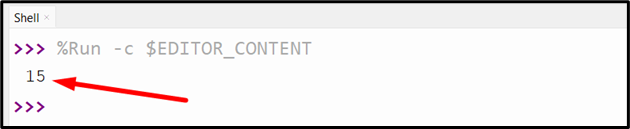According to the above results, the string has a total count of “15“.

## Method 7: Count the Characters in a String in Python Using the “reduce()” Function

The “reduce()” is a built-in function in Python that applies a function to an iterable and returns a single value. We can also apply the “reduce()” function to count the string characters.

Syntax

reduce(function, iterable, initializer=None)

In the above syntax:

• function” is a binary function that takes two arguments and returns a single value.
• iterable” is a sequence or collection that supports iteration.
• initializer” is an optional value that appears before each item of the iterable.

Example

Let’s go through the below-provided code:

from functools import reduce
string_value = "Python Tutorial"
count = reduce(lambda x, y: x + 1, string_value, 0)
print(count)

According to the above code, apply the following steps:

• The “lambda” function takes two arguments “x” and “y”, and returns “x + 1”.
• The “reduce()” function is used to apply the “lambda” function to each string character and returns the final result. The initial value is “0”.

OutputThe above output implies that the total count of the given string is “15”.

## How to Count the Specific/Particular Characters in a Python String?

To count the specific characters in a string, the following approaches can be utilized:

## Method 1: Count the Specific Characters in a String in Python Using “count()” Method

The “count()” method is used to count the total occurrences in the string or any iterable.

Syntax

list.count(value)

In this syntax, “value” corresponds to the value to search for in the list.

Example

Let’s overview the following code:

string_value = "Python Tutorial"
print(string_value.count('o'))

In the above code snippet, the “count()” function takes the specified string “o” as an argument, and returns the count of the specified character in the string.

Output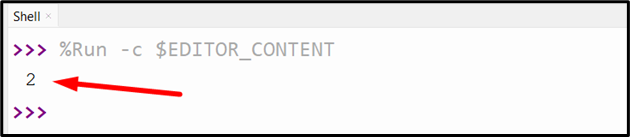Based on the above output, the total number of the specific character i.e., “o” in the string is “2”.

## Method 2: Count the Specific Characters in a String in Python Using “operator.countOf()” Function

The “ operator.countOf()” function of the “operator” module is used to count the specified characters in the string or any other iterable.

Syntax

operator.countOf(sequence, element)

In the above-given syntax:

• sequence” specifies the sequence to search for the element.
• element” indicates the element to count the occurrences of in the sequence.

Example

Here is an example code:

import operator
string_value = "Python Tutorial"
count = operator.countOf(string_value, 'o')
print(count)

In the above code, the “operator.countOf()” function takes the string and specified character as an argument, respectively, and returns the total number of occurrences of the particular character.

Output## Method 3: Count the Specific Characters in a String in Python Using the “List Comprehension” Approach

The “List Comprehension” approach is used to create the list from the existing list. This approach is used along with the “len()” function to count the specific characters in the string.

Syntax

new_list = [expression for item in iterable if condition]

Example

Following is an example code:

string_value = "Python Tutorial"
count = [i for i in string_value if i == 'o']
print(len(count))

In the above code block, the “List Comprehension” approach is applied combined with the “if” statement to return the list containing all occurrences of the matched string character. After that, the “len()” function is used to get the count of that list.

OutputThe above output shows the number of times the specific character appears in the string.

## Method 4: Count the Specific Characters in a String in Python Using “for” Loop

The “for” loop can also be used combined with the “if” statement to get the total count of the specified character in the string via iteration.

Example

Consider the below-stated code:

string_value = "Python Tutorial"
count = 0
for i in string_value:
if i == 'o':
count += 1
print(count)

According to the above lines of code, the “for” loop is used along with the “if” statement to obtain the total count of the specific character in the string.

OutputIn the above output, it can be analyzed that the total occurrence of the specific character i.e., “o” in the string is “2

## Conclusion

To count characters in a string in Python, apply the “len()” function, “Counter” class from the collections module, “dictionary comprehension”, etc. All of these methods efficiently count the characters in a string and perform text processing tasks in Python. We can also use the “for” loop, “count()” method, “List Comprehension” approach, etc. to count the specific characters in the string. This post presented various ways to count the total and specific characters in the string using numerous examples.

Source: linuxhint.com